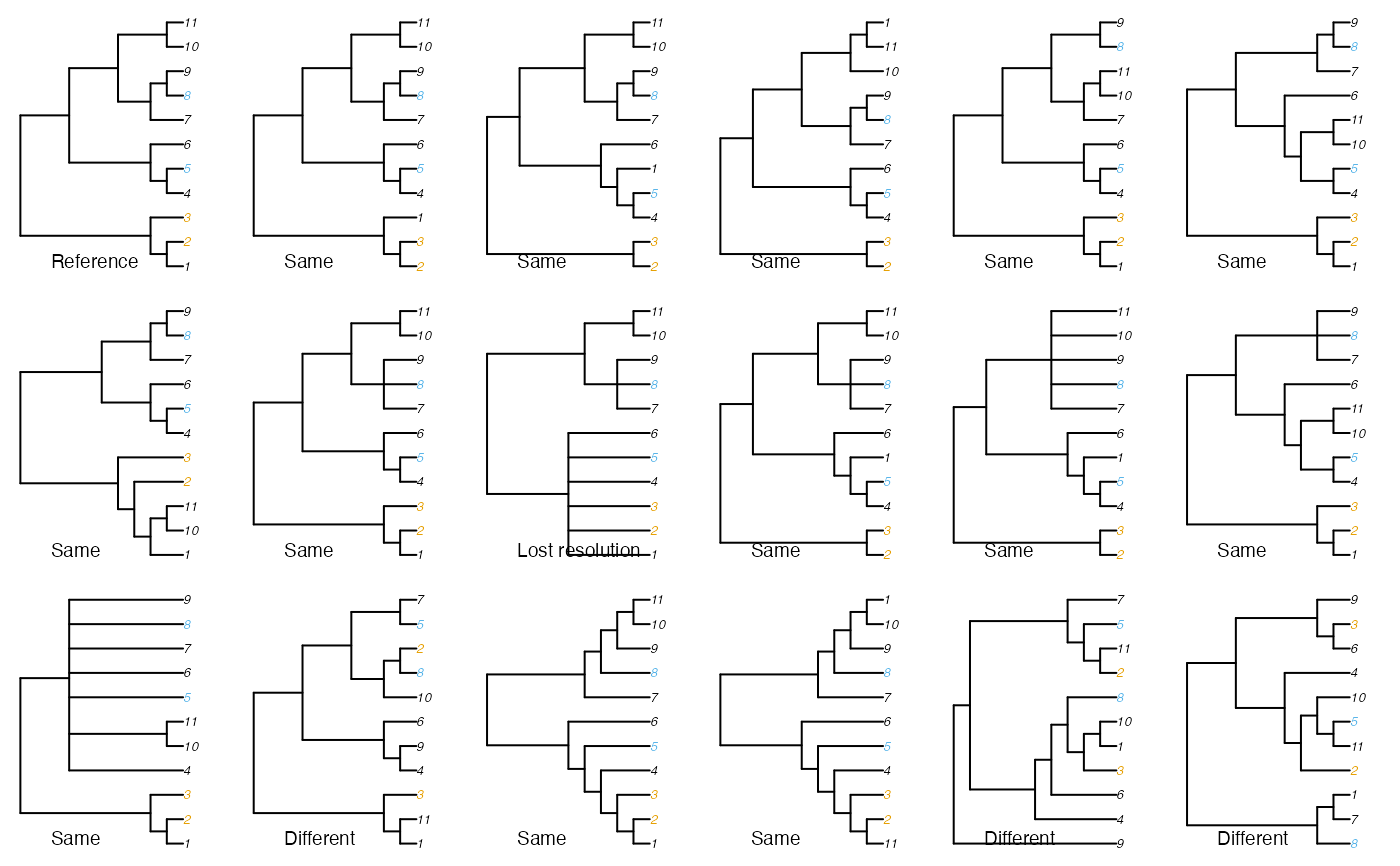Draws a tree, highlighting the members of a specified quartet in colour.

PlotQuartet(tree, quartet, overwritePar = TRUE, caption = TRUE, ...)

## Arguments

tree

A tree of class phylo, or a list of such trees. The first member of tree will be considered the 'reference' tree.

quartet

A vector of four integers, corresponding to numbered leaves on the tree; or a character vector specifying the labels of four leaves.

overwritePar

Logical specifying whether to use existing mfrow and mar parameters from par() (FALSE), or to plot trees side-by-side in a new graphical device (TRUE).

caption

Logical specifying whether to annotate each plot to specify whether the quartet selected is in the same or a different state to the reference tree.

...

Additional parameters to send to plot().

## Value

PlotQuartet() returns invisible(), having plotted a tree in which the first two members of quartet are highlighted in orange, and the second two highlighted in blue.

## Examples

data("sq_trees")

oPar <- par(mfrow = c(3, 6), mar = rep(0.5, 4))
PlotQuartet(sq_trees, c(2, 5, 3, 8), overwritePar = FALSE)par(oPar)